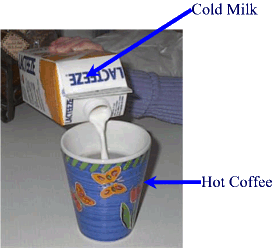#### Principle of Heat Exchange

This principle is basically a restatement of the Law of Conservation of Energy.
It says that during a process where an object or substance gains heat at the expense of another substance, the amount of heat gained by the colder substance equals the amount of heat lost by the warmer substance.
This is an idealized situation because in the real world it can only be applied if we consider a 100% closed system where no energy (heat) escapes to the outside or the surroundings.

Mathematically speaking:
Qlost = Qgained

Example:Exercise #3:
To cool down a 200 mL cup of coffee at 650C, you add 15.0 mL of milk at 10.0 0C.
What is the final temperature of  the coffee?
The specific heat capacity of milk is 3700 J/K.Kg, the specific heat capacity of  coffee is 4200 J/K.Kg,  [For coffee and milk assume that the densities are the same as water. i.e.: D =1.0 g/mL]